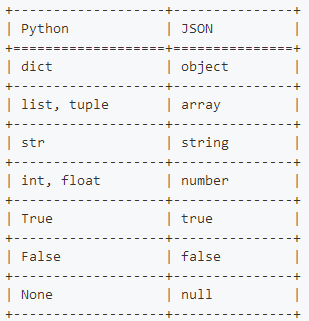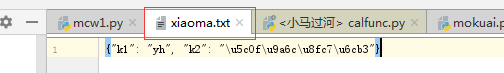python json模块使用详情

#json。数据交换用到json文件。json是特殊的字符串。访问网站，返回的就是json

对象表示为键值对
数据由逗号分隔
花括号保存对象
方括号保存数组

2、注意点

json是字符串
json中不存在元组。序列化元组之后元组变列表；不能是集合，序列化集合报错。序列化支持类型可以进Python官方文件介绍里面有介绍。

import json
tup=(1,2,3)
a=json.dumps(tup)   #json中不存在元组。序列化元组之后元组变列表
print(a)
--------------结果：
[1, 2, 3]
[1, 2, 3]

import json
mset={1,2,3}      #不能是集合，序列化集合报错。
print(json.dumps(mset))
-------------结果；
o.__class__.__name__)
TypeError: Object of type 'set' is not JSON serializable

d = {
'a': 123,
'b': {
'x': ['A', 'B', 'C']
}
}

json格式

{
"a": 123,
"b": {                #必须是双引号（因为java等其它语言有使用双引号表示字符串，单引号不表示字符串）
"x": ["A", "B", "C"]
}
}

3、json使用(常用)：

JSON(JavaScript Object Notation, JS 对象标记) 是一种轻量级的数据交换格式。JSON的数据格式其实就是python里面的字典格式，里面可以包含方括号括起来的数组，也就是python里面的列表。

1）# 序列化，将python的值转换为json格式的字符串。序列化json.dumps() True变true了。序列化
import json
v = [12,3,4,{'k1':'v1'},True,'asdf']
v1 = json.dumps(v)
print(v1,type(v1))
--------------结果；
[12, 3, 4, {"k1": "v1"}, true, "asdf"] <class 'str'>

import json
v2 = '["mcw",123]'
print(type(v2))
print(v3,type(v3))
-----------------结果；
<class 'str'>
['mcw', 123] <class 'list1）字典序列化：
import json
dic={"name":"mcw","age":18}
xu=json.dumps(dic)
print(xu,type(xu),type(dic))
--------------结果：
{"name": "mcw", "age": 18} <class 'str'> <class 'dict'>

）列表序列化与反序列化：
import json
li=[1,2]
xu=json.dumps(li)
print(xu,type(xu),type(li))
print(fx,type(fx))
------------结果：
[1, 2] <class 'str'> <class 'list'>
[1, 2] <class 'list'>

3）字符串序列化与反序列化:
import json
mcwstr="xiaoma"
xu=json.dumps(mcwstr)
print(xu,type(xu),type(mcwstr))
print(fx,type(fx))
---------------结果；
"xiaoma" <class 'str'> <class 'str'>
xiaoma <class 'str'>

4）整型序列化与反序列化
import json
mcwint=2
xu=json.dumps(mcwint)
print(xu,type(xu),type(mcwint))
print(fx,type(fx))
---------------结果：
2 <class 'str'> <class 'int'>
2 <class 'int'>

5）浮点型序列化与反序列化
import json
mcwfloat=2.03
xu=json.dumps(mcwfloat)
print(xu,type(xu),type(mcwfloat))
print(fx,type(fx))
--------------------结果：
2.03 <class 'str'> <class 'float'>
2.03 <class 'float'>

6）布尔型序列化与反序列化：
import json
mcwbool=True
xu=json.dumps(mcwbool)
print(xu,type(xu),type(mcwbool))
print(fx,type(fx))
------------------结果：
true <class 'str'> <class 'bool'>
True <class 'bool'>

7）None序列化与反序列化
import json
mcwnone=None
xu=json.dumps(mcwnone)
print(xu,type(xu),type(mcwnone))
print(fx,type(fx))
---------------结果；
null <class 'str'> <class 'NoneType'>
None <class 'NoneType'>

4、json序列化过程中的中文显示

import json
v = {'k1':'alex','k2':'魔降风云变'}
print(json.dumps(v))
val = json.dumps(v,ensure_ascii=False)
print(val)
--------------------结果：
{"k1": "alex", "k2": "\u9b54\u964d\u98ce\u4e91\u53d8"}
{"k1": "alex", "k2": "魔降风云变"}

import json v
= {'k1':'yh','k2':'小马过河'} f = open('xiaoma.txt',mode='w',encoding='utf-8') #文件不存在就会生成 val = json.dump(v,f) print(val) f.close() ----------------结果： None #dump将内容序列化，并写入打开的文件中。

import json f
= open('xiaoma.txt',mode='r',encoding='utf-8') data = json.load(f) f.close() print(data,type(data))
---------------结果:
{'k1': 'yh', 'k2': '小马过河'} <class 'dict'>1）https://www.cnblogs.com/tjuyuan/p/6795860.html
2）http://www.runoob.com/python/python-json.html
3）https://www.jb51.net/article/139498.htm

posted @ 2019-04-17 17:09  马昌伟  阅读(12686)  评论(0编辑  收藏  举报